# Earth curvature: Differences with Sphere/Ellipsoid/Geoid models for Visibility etc

#### DarkStar

##### Active Member
It is time to revisit the ellipsoid question as mentioned by @Rory here and Mick's thoughts here. And Rory linked to this awesome page with Earth ellipsoid and geoid radius calculator integrated and explains the math behind it: SummitPost

So here is my brain dump in the hopes that we can collaborate on this and at least all understand the situation better, even if we don't update the calculator itself.

History:

So based on the now Infamous Balaton Lake Debacle (IBLD) a discussion thread ensued in another forum and one of the posters again mentioned the Ellipsoid and posted some calculations which showed ~1.67 meters *per* kilometer over this Lake - which is greater than the curvature. I never thought it would matter that much so I hadn't really focused on this specific problem before in my calculations. But it does matter and it can matter a whole lot especially as the direction of observation goes more North/South (the earth radius is increasing as you move South, and at some angle away from South you get some fraction of that effect).

And I am *NOT* talking about E/W observations following a line of Latitude which get smaller and smaller as you move North -- Latitude lines are NOT great circle lines - so they are NOT the path under a line of sight (except at the equator. You have to get that image out of your head. When we talk about point A to point B you have to intersect a plane with the center of the Earth and follow the surface of that intersection which doesn't follow lines of latitude (again, except at the equator).

Ok, so here is the scenario I looked at...

One of my first forays into the Flat Earth world was looking at the view from Olcott, NY toward Toronto and the famous CN tower. It was obvious that observer height mattered so I first derived the equations for this calculation (Mick's Metabunk calculator was more barebones at that time but I DID find his early GeoGebra calculator, which also got me interested in GeoGebra & MetabunkSo I got the distances, estimated the observers height, checked the elevation profile from Google Earth, AND I even used the rechneronline calculator to get the approximate ELLIPSOIDAL Earth radius at this point. I plugged in my numbers for no refraction and 7% refraction and got the typical, fairly reasonable results.

Which, frankly, I think is usually sufficient to show that the views we see are compatible with a curved Earth.

HOWEVER, upon revisting this due the oddness of the IBLD results (because the FEW observations we can make from that mess really did NOT fit the spherical model - they didn't fit a flat 'model' *cough* either. Refraction didn't really explain it either) I started seeing some significant impact on the results -- they fit much better once you accounted for the significant delta in Earth radius along that 6 kilometer run (for example, instead of the laser having a slight downward slope it actually had a slight upward slope, but "MSL" was also rising under you).

So I revisted the Toronto view and here is what I found:

Elevation, Radius(includes Geoid), & Geoid in meters, lat/long in decimal (neg = S/W)
CN Tower: 43.641729 -79.384076 / E=81 / R=6367960.569 / G=-35.626
Olcott Pier: 43.339961 -78.717597 / E=75 / R=6368073.305 / G=-35.312
Olcott Pier Geoid is 112.736m HIGHER than CN Tower - 6m elevation difference + 3.5m viewer = ~110m higher!

All of sudden your expected hidden height is then maybe significantly less than the expected ~255 meters at 63.6km. But I'm not sure yet how to actually calculate the view. Can I count it as a 110m high observer? And if I then plug that into a spherical calculator I don't think that is going to give me a solid answer.

Mick suggested in his post that you can just scale the results... I'm not sure. So what I propose is that we tackle nailing this down so that all of our predictions can be more accurate. I do think these findings suggest that the ellipsoidal difference will be significant in some measurements (especially for observations at those mid-latitudes running more N/S).

My next approach is to the take the equation for a circle and derive the horizon equation from it - and then do the same for the ellipse, that is -- get a an equation I can plug the observation elevation into and get the horizon distance back out that fits to the ellipse. That should be pretty easy -- I just need to find the time (instead I thought I would share my findings so far).

When you have an elevation (especially from Google Earth) that is giving you the Orthometric height as shown below, so to really compare two Orthometric heights you need the Geoid, and the Geoid is a delta from the Ellipsoid.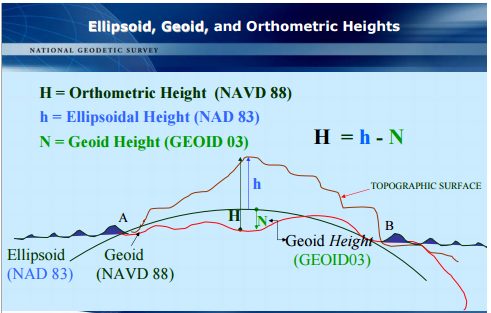Here is how you get the radius at some latitude from the WGS-84 ellipsoid:

f=1/298.25722356 << given by the model ('flattening')
a=6378137 << given by the model
b=a-(a*f) << computed
θ = geocentric latitude (tan θ = (b²/a²) tan ϕ)
ϕ = geographic latitude
R(θ) = √(((a²×cos(θ))² + (b²×sin(θ))²) / ((a×cos(θ))² + (b×sin(θ))²))

Latitude ϕ also needs to be the correct 'kind' of latitude, I assume Google Earth gives you geographic latitude so you have to rescale it, (b²/a²) ~ 0.9933 -- so it's a slight correction to the slope but 42° becomes 41.81°

From there the Geoid height is hard to get (you have to download the database and do some complex computations based on spherical harmonic expansions to get the height for any given point).

However, they do have a 'Geoid Height' file you can download and just do some interpolation (which should be more than accurate enough for what we're doing). I think if we can JUST get the ellipsoid model working we will be in much better shape. That's my next step anyway.

Handy publication notes/reference:
http://www.ngs.noaa.gov/GEOID/PRESENTATIONS/2007_02_24_CCPS/Roman_B_PLSC2007notes.pdf
http://www.esri.com/news/arcuser/0703/geoid1of3.html
http://earth-info.nga.mil/GandG/wgs84/gravitymod/egm2008/egm08_wgs84.html
http://earth-info.nga.mil/GandG/wgs84/gravitymod/

UPDATE: I did the same thing for the famous 'Bedford level' area between the bridge at Welney and the bridge near the Welches dam. Because this is England I did it in feet (actually feet are slightly more fine-grained).

Bedford level: 31,688' distance (WGS84 curvature distance)
Welney bridge: 52.519628° 0.250938° / E=12' / R=20881755.992' / G=154.577'
Welches dam: 52.451411° 0.162850° / E=11' / R=20881837.357' / G=155.071'
delta R = 81.365'

So this means that level ground across that 6 mile difference is 81' further from the center of Earth mass at one end than the other. That does NOT mean there is an 81' slope - this is the equipotential gravity level line. The elevation is harder to tell from this data but appears to be approx 1 foot but that could just be the ground around the water, not necessarily the water level itself. The Geoid shift seems to be about 6 inches (again, higher at Welches which is further south).

This page seems to have some of the math we need (start at 'Radius of Curvature Derivation - General Case'):

http://www.cohp.org/local_curvature.html

Last edited:

#### Mick West

Staff member
Mick suggested in his post that you can just scale the results... I'm not sure.

I no longer think this is exactly true.

A line from the viewer through the center of a sphere is perpendicular to the surface. However if you scale that then the line is no longer perpendicular to the surface.

So you've got two competing "height" values here.

A) The height of a line drawn from the viewer towards the center of the spheroid to where it intersects the surface
B) The height a line drawn from the viewer to the closest point on the spheroid

Now B is what people would actually be measuring, but A is what you would get if you just transform the sphere calculations.

#### DarkStar

##### Active Member
I no longer think this is exactly true.

Yeah, it is suggested elsewhere that the problem isn't analytically solvable except by assuming certain approximations.

http://www.cohp.org/local_curvature.html

seems to be a good primer but doesn't seem to answer the "distance to horizon" question directly, but give a different formula for the radius calculation.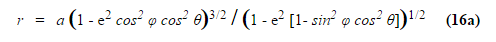I haven't played with that version yet but suspect it's close to the other one I posted.

There obviously isn't a single 'distance to the horizon' on a ellipsoid so we need to know which direction we are looking as an additional parameter.

I'm trying to visualize what this "looks like" to an observer.

Another version of this equation from summitpost, where Z is orthographic height, G is Geoid height:

√[(a⁴ cos²(ϕ)+ b⁴ sin²(ϕ))/(a² cos²(ϕ) + b² sin²(ϕ)) + 2(Z + G)√[a² cos²(ϕ) + b² sin²(ϕ)] + (Z + G)²]

Fun things to play around with laterOh -- a quick aside for Mick, looks like a 122 degree Rectilinear lens is in the works. Looks pretty amazing.

Last edited:

#### Mick West

Staff member
My next approach is to the take the equation for a circle and derive the horizon equation from it - and then do the same for the ellipse, that is -- get a an equation I can plug the observation elevation into and get the horizon distance back out that fits to the ellipse. That should be pretty easy -- I just need to find the time (instead I thought I would share my findings so far).

I was thinking I might try doing a computational approach, that way you could simply plug in different model (sphere, various ellipsoids, various geoids) and the higher level code would all be the same.

The model would just return the"radius" (distance to center) for a given set of polar coordinates. (or maybe a point [x,y,z]). From that you can calculate the tangent plane, could do a binary search between the two ground points A and B, to find a point C (the horizon) at which the rate of change of the angle AVC become zero. (V being the view point above A). Or just when the distance of V from the tangent plane at C is zero (or crosses zero).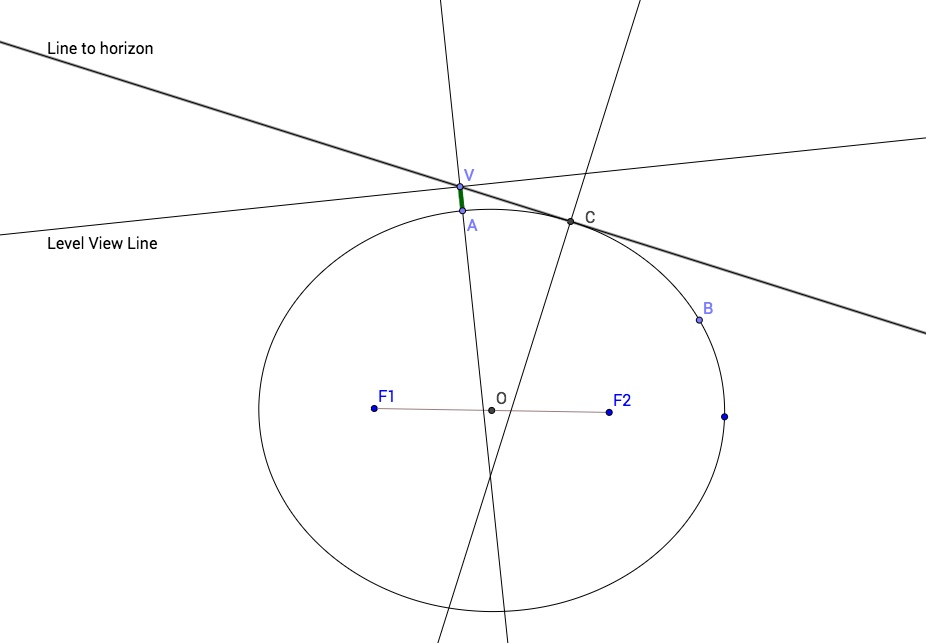What is somewhat confusing here, as before, is that vertical lines do not pass through the center of the ellipsoid.

#### Mick West

Staff member
Latitude ϕ also needs to be the correct 'kind' of latitude, I assume Google Earth gives you geographic latitude so you have to rescale it, (b²/a²) ~ 0.9933 -- so it's a slight correction to the slope but 42° becomes 41.81°

Interesting, I'd always assumed the latitude was essentially the angle between a surface point, the center of the earth, and the equator (geocentric). But it seems the default is geodetic "the angle between the normal and the equatorial plane", which makes sense.

Google Earth and GPS use WGS84 geodetic latitude.

Just a reference so I know which way x, y and z go: Z is the Earth's axis, with north being positive. X comes out at the zero meridian, and Y comes out in the east.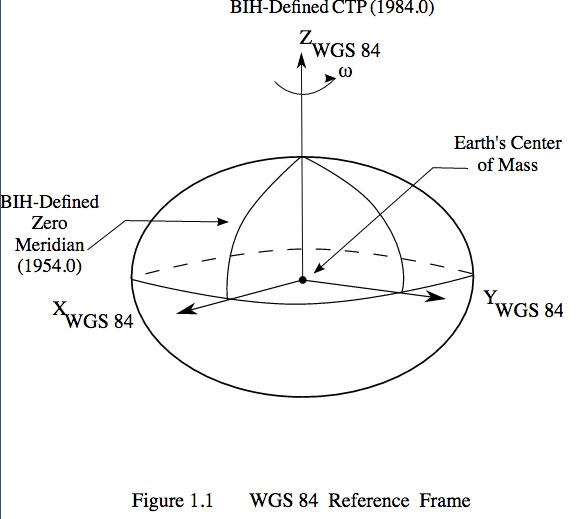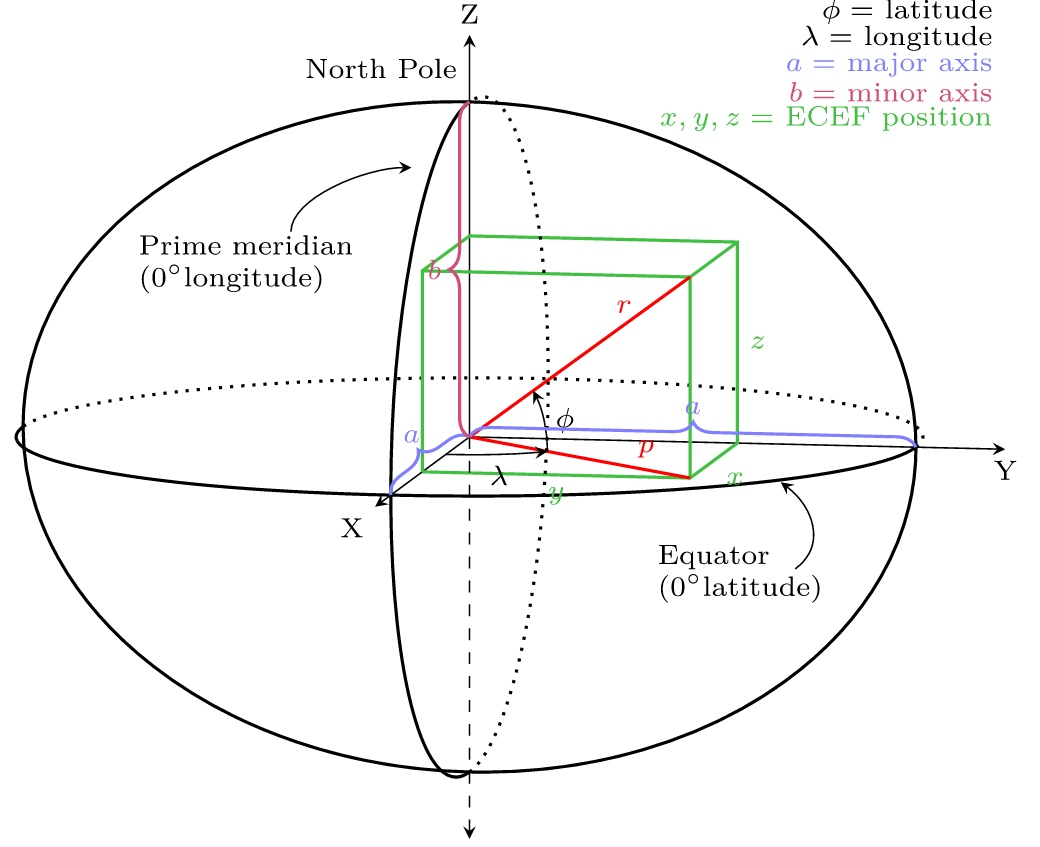Last edited:

#### Mick West

Staff member
I did a little work on this, got as far as calculating the horizon using WGS84. The algorithm was basically:

Given two points, A and B defined by latitude and longitude, and a view point V that is h meters above A, then use a binary search to find the point C between A and B where the angle V->C->D is 90 degrees. (D is an arbitrary point 100m above C, which gives you the normal vector).

Or in code:
Code:
``````var steps = 300;

// A is the point on the surface
var A = vecA(LLAToECEF(latA,lonA,0));
// B is the point on the surface at the target
var B = vecA(LLAToECEF(latB,lonB,0));

// h is the height above the surface in meters of the viewpoint, so V is the viewpoint
var V = vecA(LLAToECEF(latA,lonA,h));

// Binary search approach
// we are stepping from A to B, so just pick a point C in the middle
// if it's positive, then that's the new A
// if it's negative, then the new B
// repeat until things converge, or for a number of steps to get sufficent accuracy
// also need to check if B is positive, meaning the horizon is past B
// TODO: use the real lat/long midpoint, not an interpolation

var A2B = new THREE.Vector3();
A2B.subVectors(B,A);
console.log("AB length = "+A2B.length());

var last_length = 0;
var latC, lonC;
for (var i=0;i<steps;i++) {

// get midpoint
latC = (latA+latB)/2;
lonC = (lonA+lonB)/2;
var C = vecA(LLAToECEF(latC,lonC,0));
var D = vecA(LLAToECEF(latC,lonC,100)); // D is a point 100m above C

var V2C = new THREE.Vector3();
V2C.subVectors(C,V);	// C-V

// get the surface vector C->D
var C2D = new THREE.Vector3();
C2D.subVectors(D,C);
var angle = V2C.angleTo(C2D)-Math.PI/2;

if (angle > 0) {
latA = latC;
lonA = lonC;
}
else
{
latB = latC;
lonB = lonC;
}

var length = V2C.length()
console.log ("V2C.length = " + length);
if (length == last_length)
break;
last_length = length;

}
console.log("Horizon point at: "+Math.degrees(latC)+","+Math.degrees(lonC));``````

For A = (46.949220, 17.889290), B = (46.904430, 17.934170), and a view height of 1m, this gives me the horizon at (46.922735848790516,17.915827367856256), with a straight line length from V to C of 3570.9772808189873.

Putting in the straight line AB distance of 6.039559142877003 into the calculator gives a horizon distance of 3569.59, so about 1.387m difference in the distance to the horizon.

Later I'll try the "drop" from the level plane at A or V.

The WGS84 conversion function comes from:
https://github.com/lakowske/ecef-projector/blob/master/index.js

Last edited:

#### Mick West

Staff member
The "drop" calculation is quite simple. Given two points on the surface A and B, and the unit normal vector at A (n), then the drop of B below the level plane at A is AB.n (where AB is the vector from A to B, "." is the dot product.

Adding to the code above, we calculate the unit normal from AV, then just dot it with AB
Code:
``````var A2V = new THREE.Vector3();
A2V.subVectors(V,A);
A2Vn = A2V.clone().normalize();

var drop2 = -A2Vn.dot(A2B);``````

For our test case above, this gives a drop of 2.860459556m, whereas the sphere drop for the same 6.03955914 distance is 2.8626811m.

So using the WGS84 ellipsoid, the drop over 6KM is only 2.2mm different to the value calculated using a sphere. Unless I got somethings wrong.

#### Mick West

Staff member
I modified the code to allow for dropping in an arbitrary model for LLA to ECEF (i.e. Lat, Long, Altitude to X,Y,Z), and used the function:

Code:
``````function LLAToECEF_Sphere(latitude, longitude, altitude) {

var a	= 6371000;  // using the standard 6371km
var X = (a + altitude) * Math.cos(latitude) * Math.cos(longitude);
var Y = (a + altitude) * Math.cos(latitude) * Math.sin(longitude);
var Z = (a + altitude) * Math.sin(latitude);

return [X, Y, Z];
}``````

Which just models a sphere of radius 6371km

For our approximately 6km test case with both WGS84 and Sphere models we have:

WGS84:
AB length = 6039.559142877003
V2C.length = 4829.142555651498 (horizon distance)
drop2 = 2.8604607901052077
Calculator drop = 2.862681120634079

Sphere:
AB length = 6034.888881026446
V2C.length = 4827.273810959408
drop2 = 2.8582556628293787
Calculator Drop = 2.858255530707538

AB length is the cartesian distance between points A and B as calculated using the model (i.e. A and B are converted into XYZ coordinates, then the length of the vector between them is used)

V2C.length is the distance from the viewpoint V to the point C which is the horizon point found by the binary search. i.e. it's the distance to the horizon viewed from V looking towards B.

drop2 is the perpendicular distance of point B from the plane defined by point A and the surface normal at A.

Calculator drop is the value of drop the simple sphere based calculator returns when given the distance as AB length.

Distances are in meters. So for the same latitude and longitude points A and B, the distance between the points is 4.67m more on the WGS84 ellipsoid model, and the distance to the horizon is 1.87m more . However the the drop from the level plane at B only increases by 2.2mm

Hence there's really no benefit to using WGS84 instead of a spherical model to calculate "drop" and "hidden" values. Refraction effects will vastly outweigh the differences between the models.

Last edited:

#### Rory

##### Closed Account
Just revisiting this and wanted to say, damn, this is a good thread.